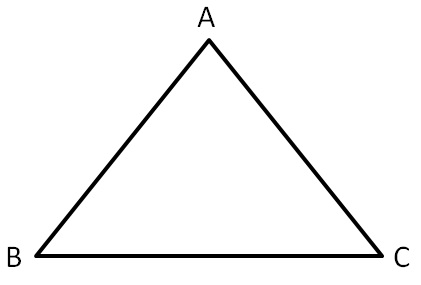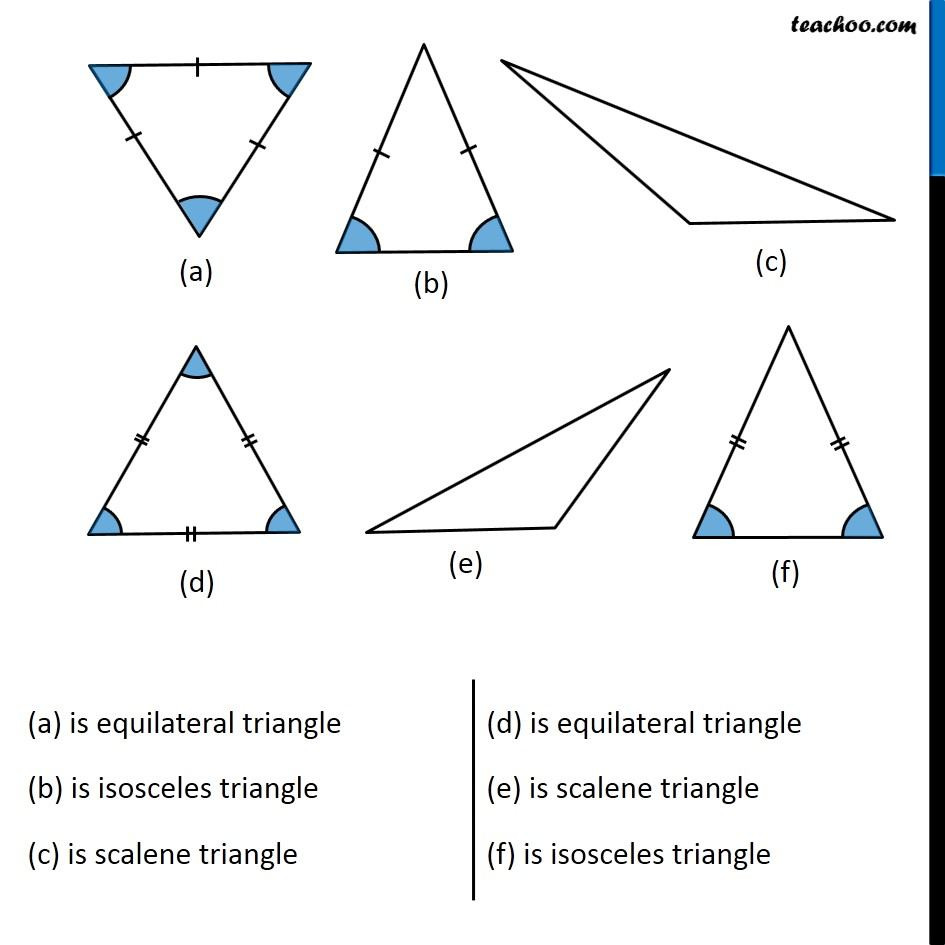Classifying triangles

Chapter 5 Class 6 Understanding Elementary Shapes
Concept wiseNow, in ∆ABC,

there can be 3 cases

• All sides are different
• Two sides are same
• Three sides are same.

If all three sides are different, it is a scalene triangle.

If two sides are equal, it is an isosceles triangle.

If all three sides are same, it is an equilateral triangle.Get live Maths 1-on-1 Classs - Class 6 to 12

### Transcript

(a) is equilateral triangle (b) is isosceles triangle (c) is scalene triangle (d) is equilateral triangle (e) is scalene triangle (f) is isosceles triangle